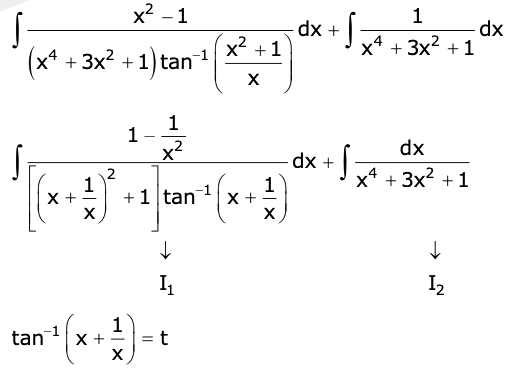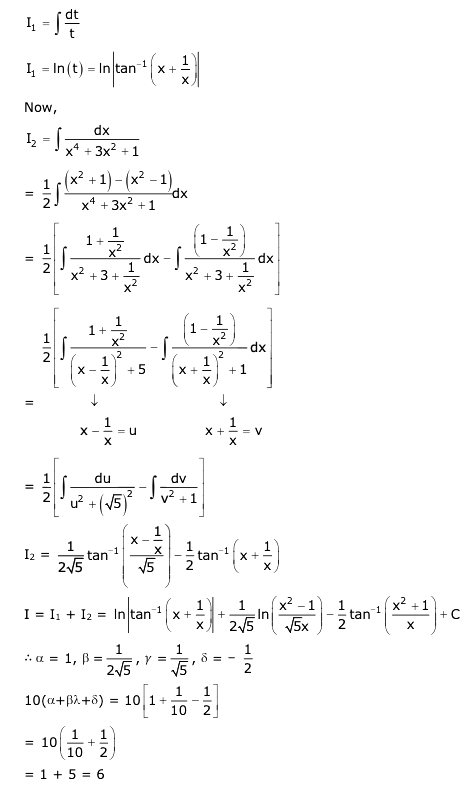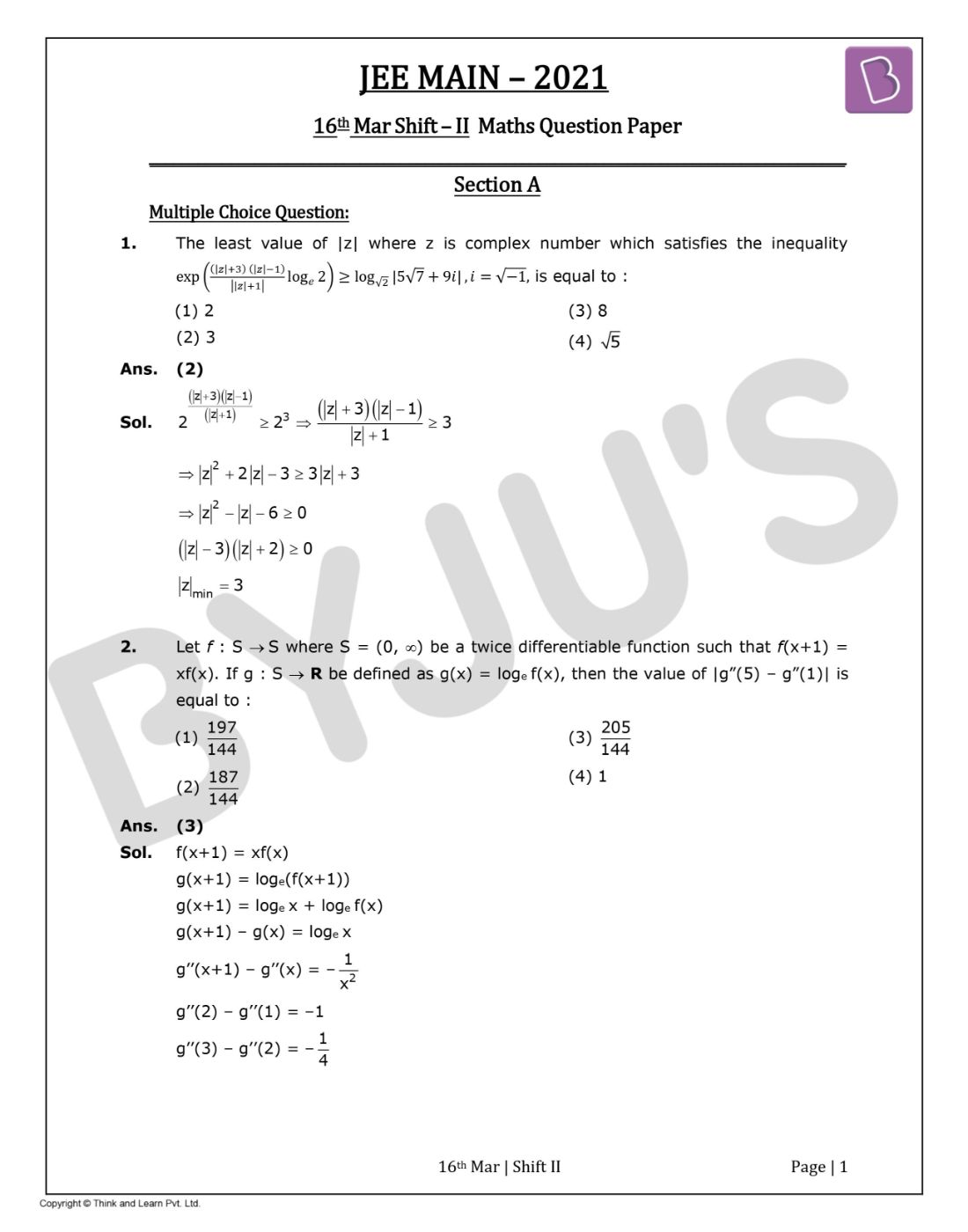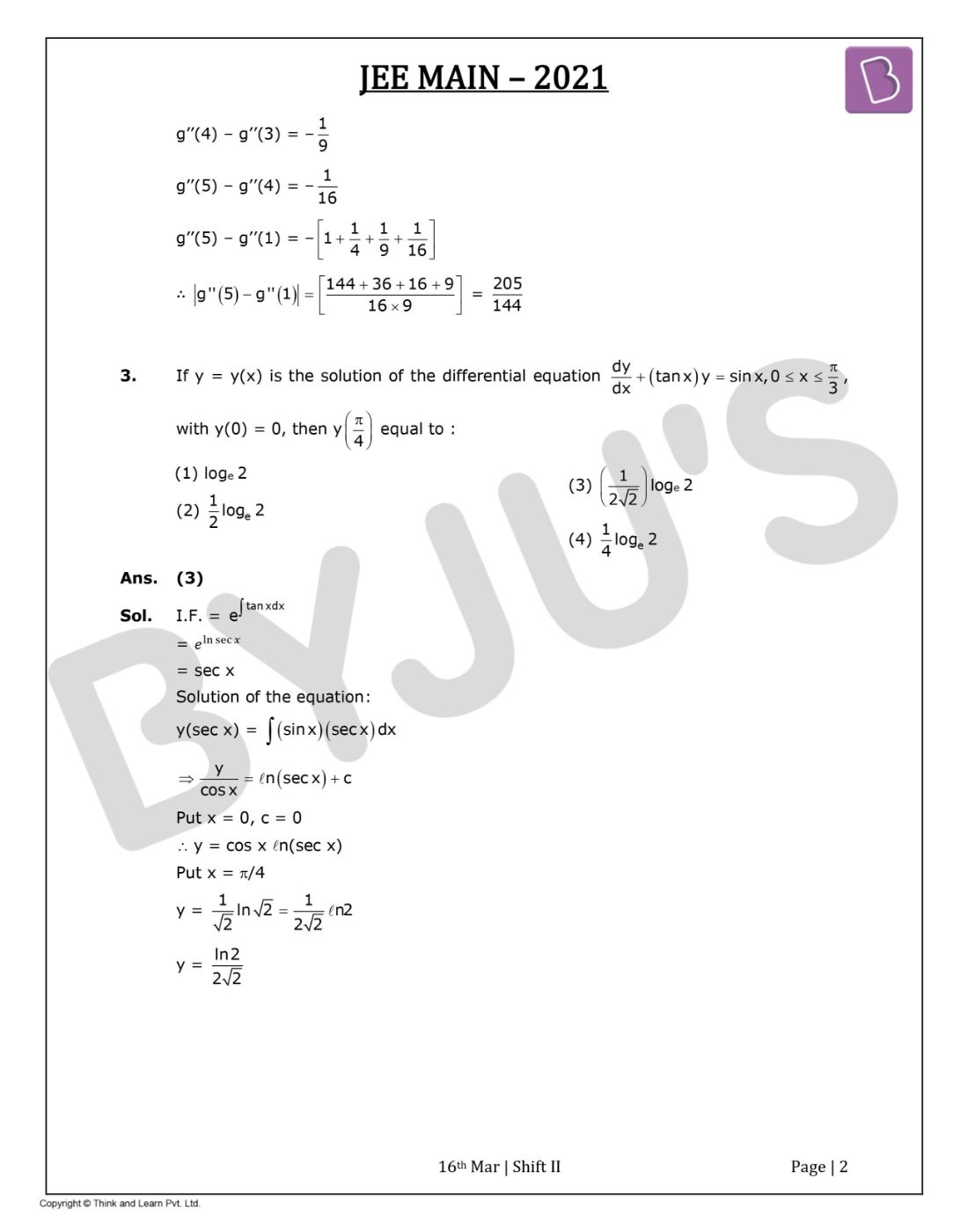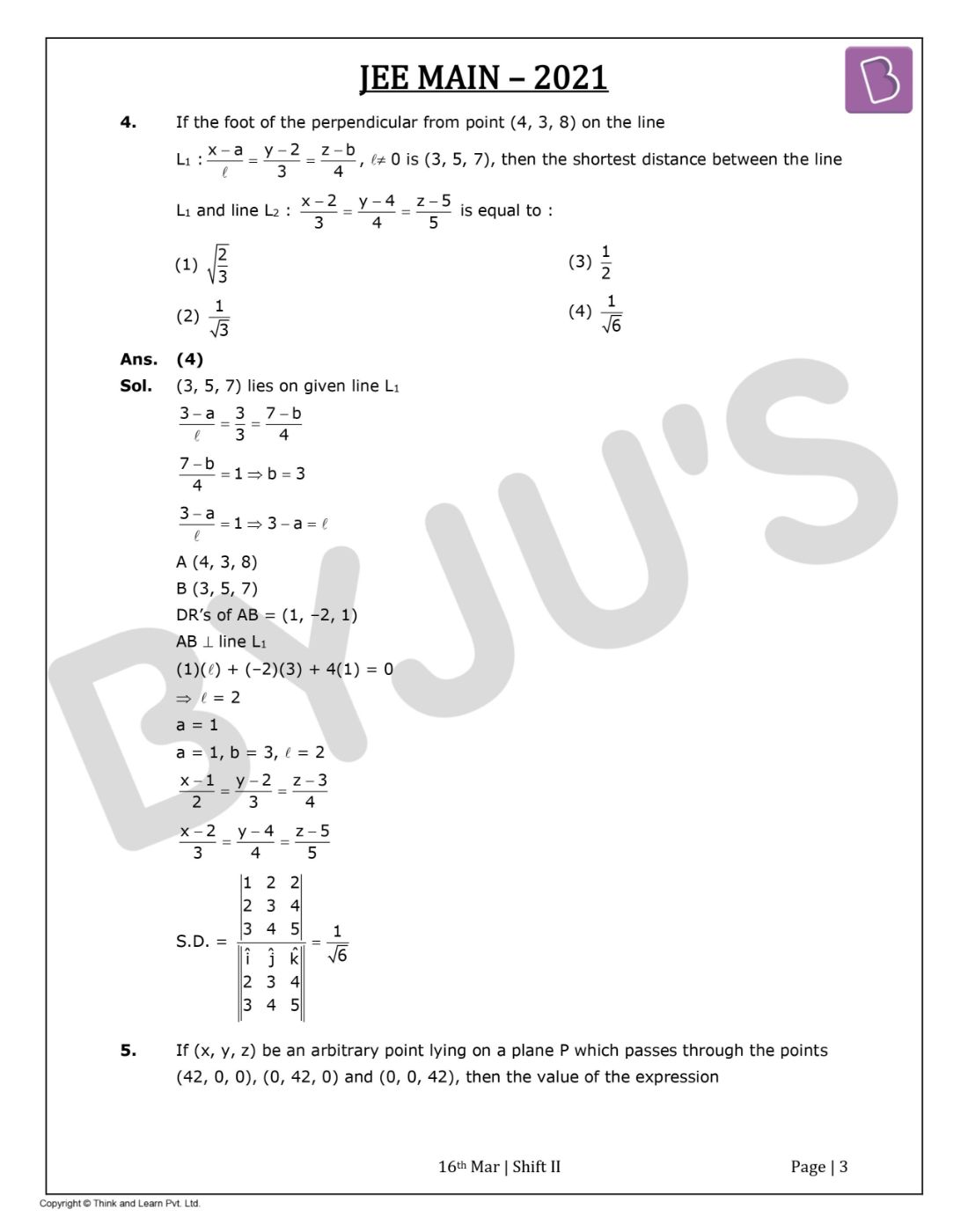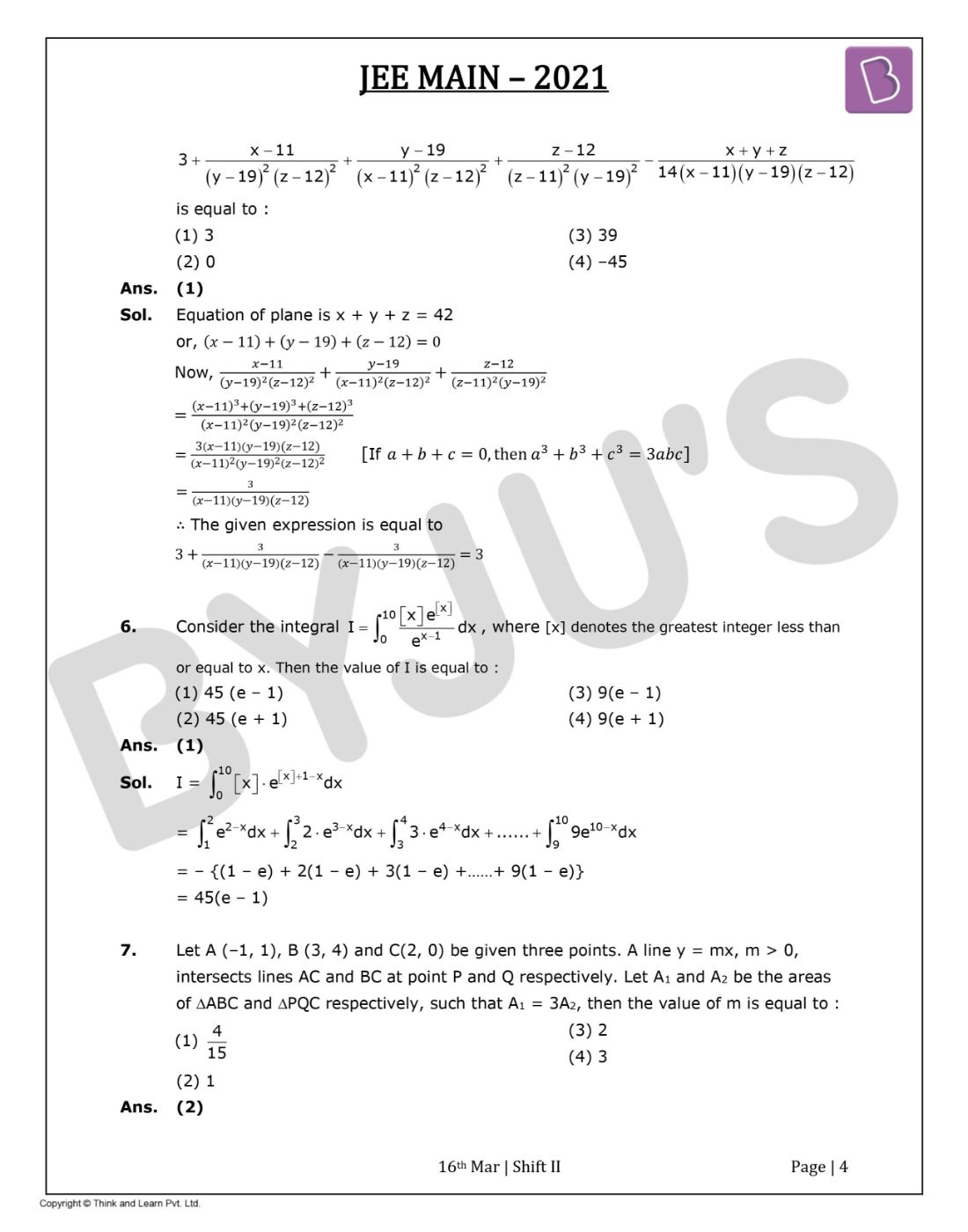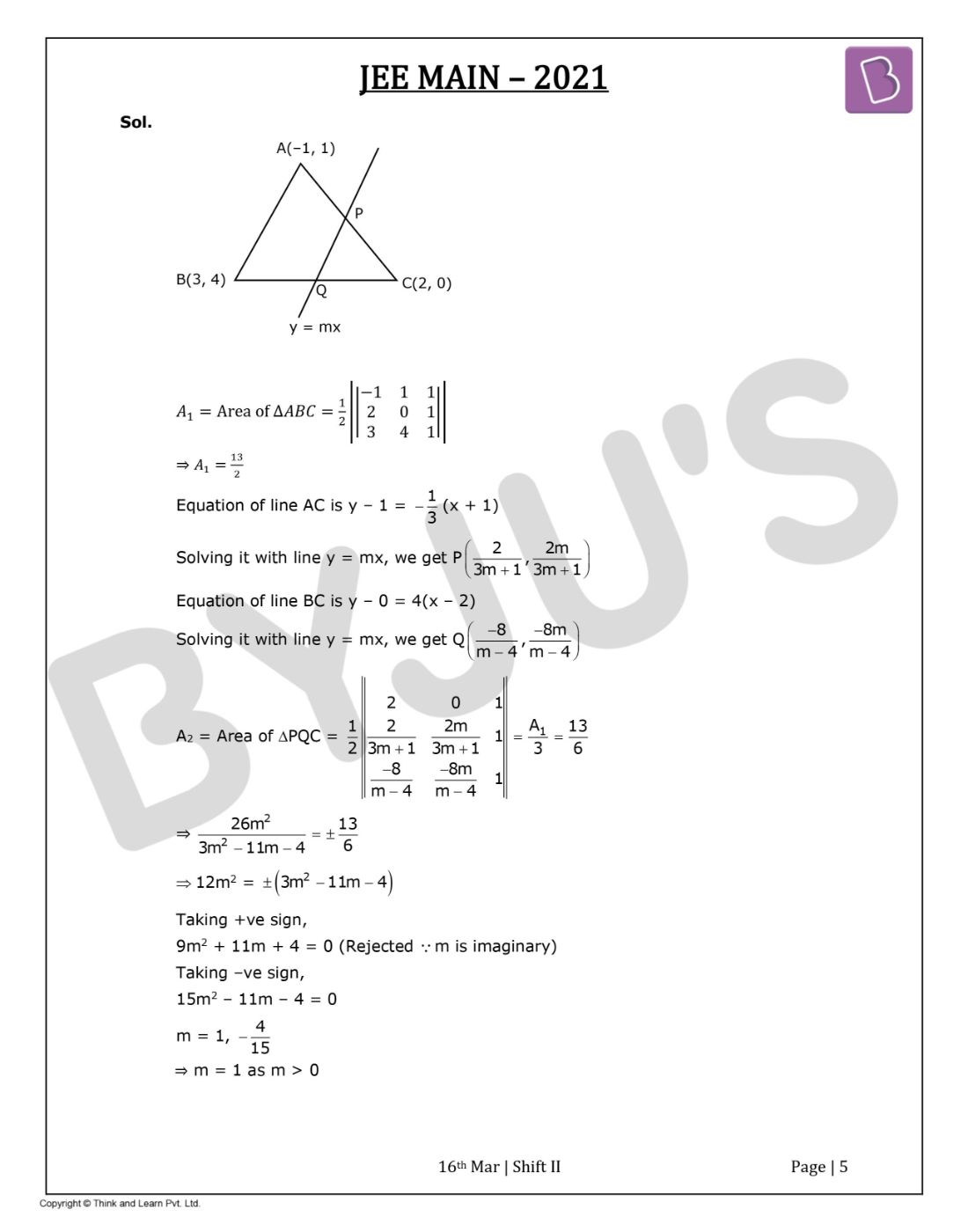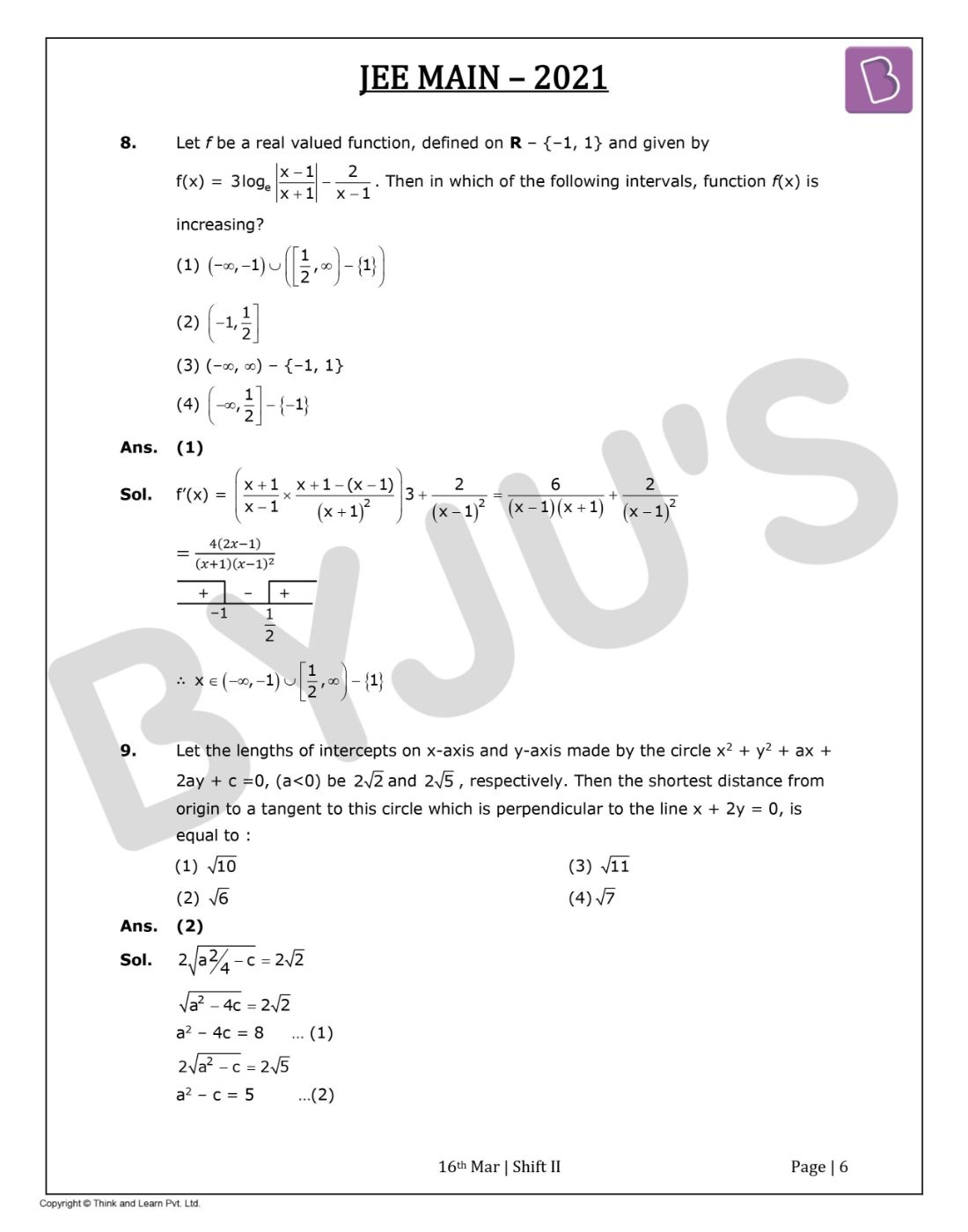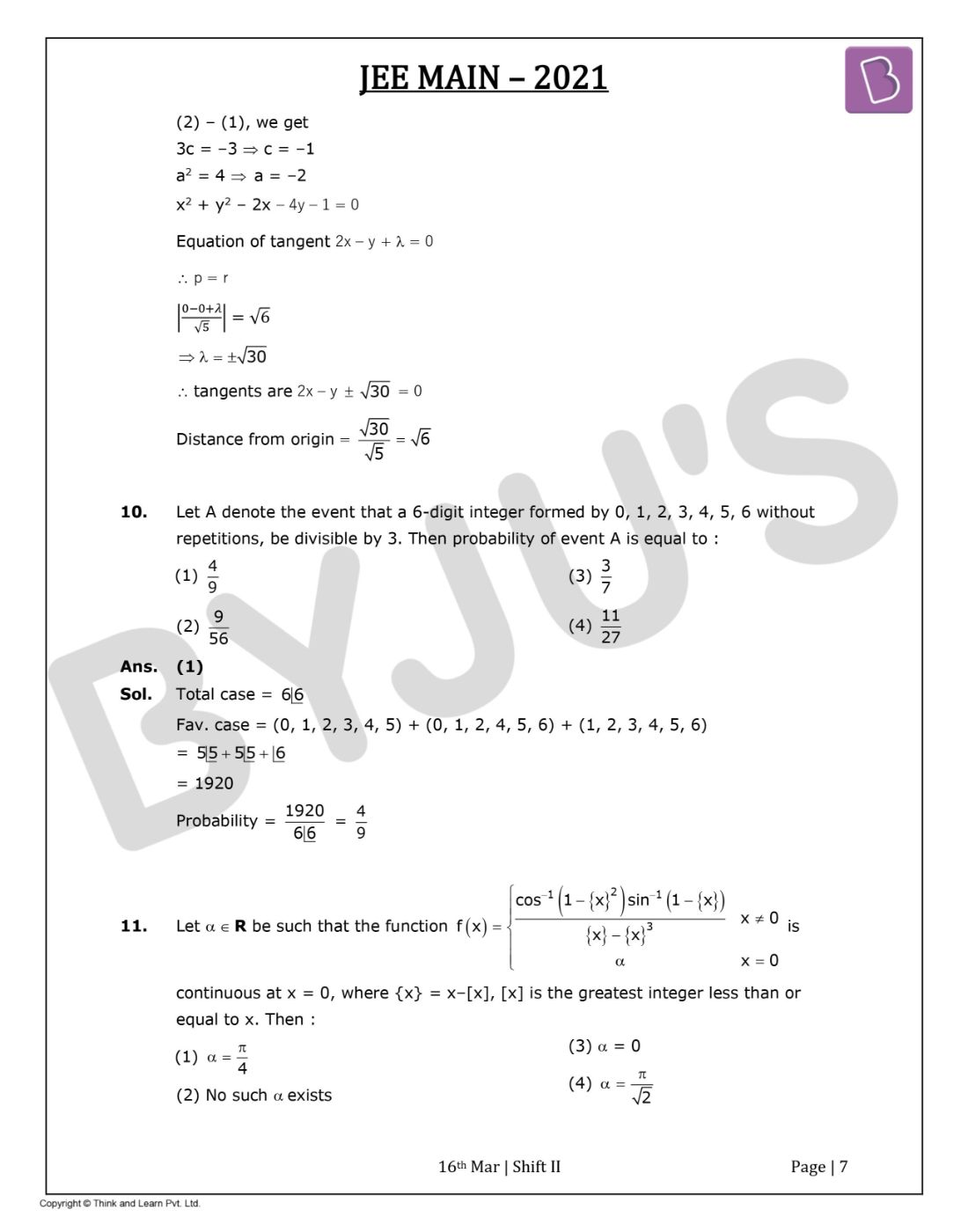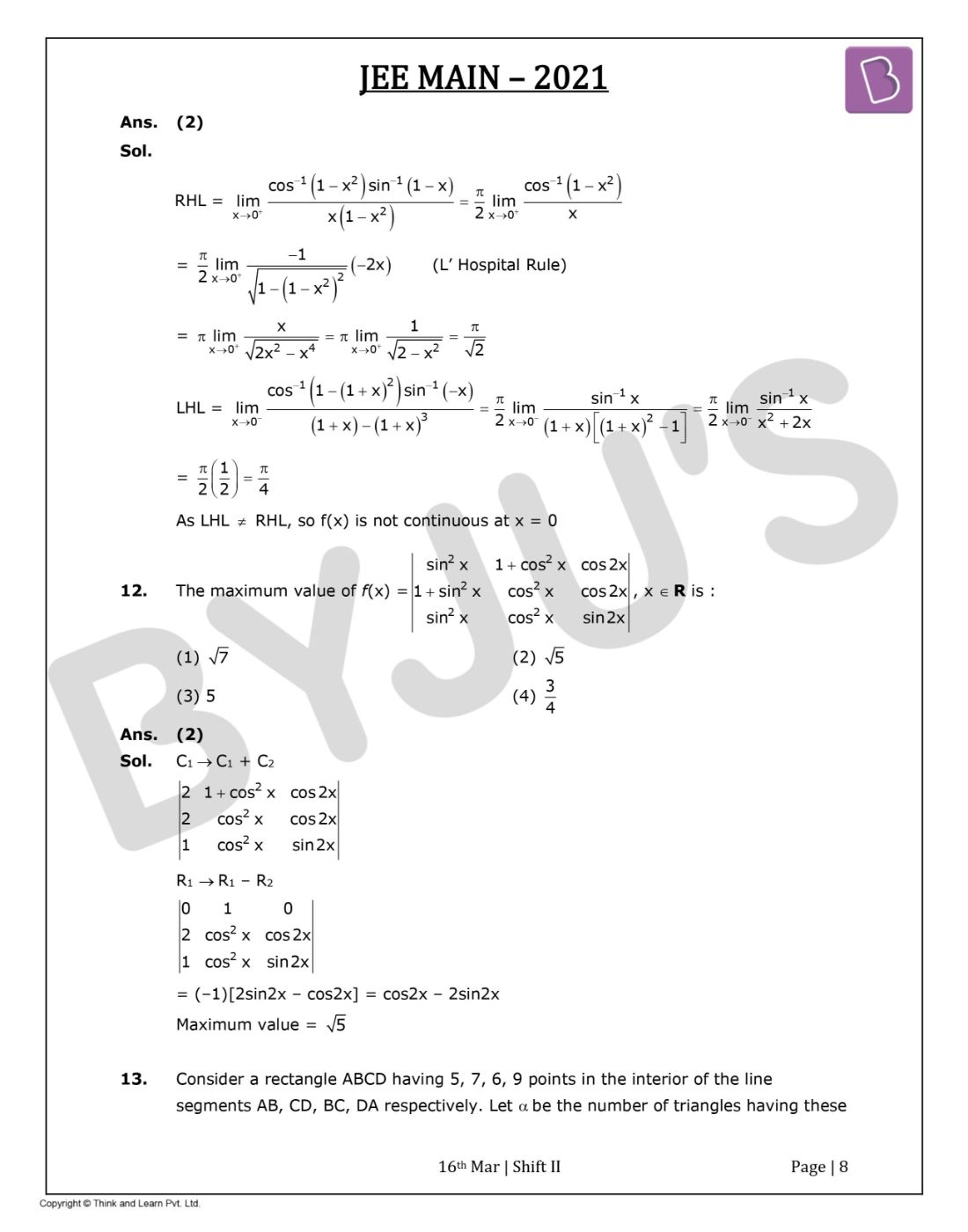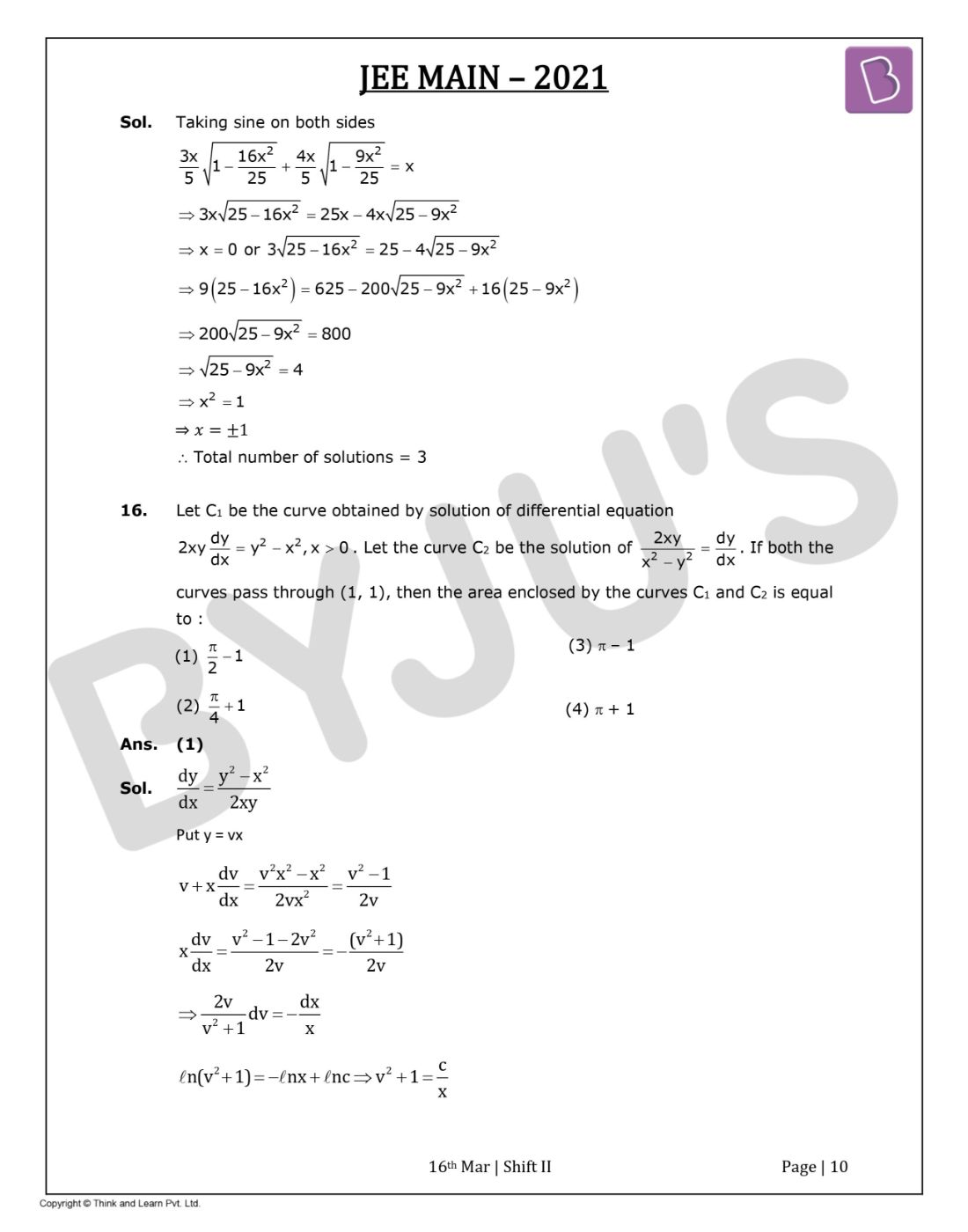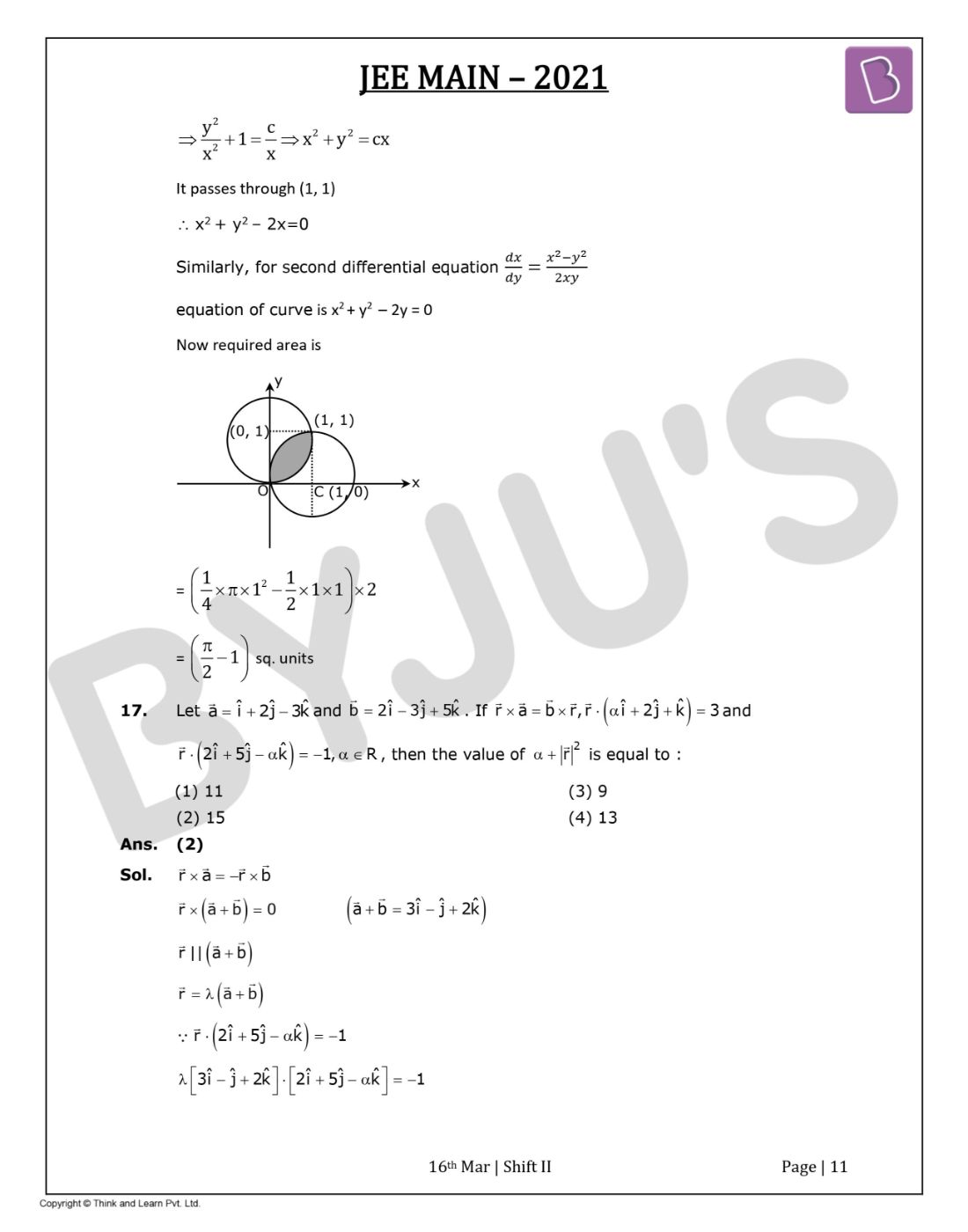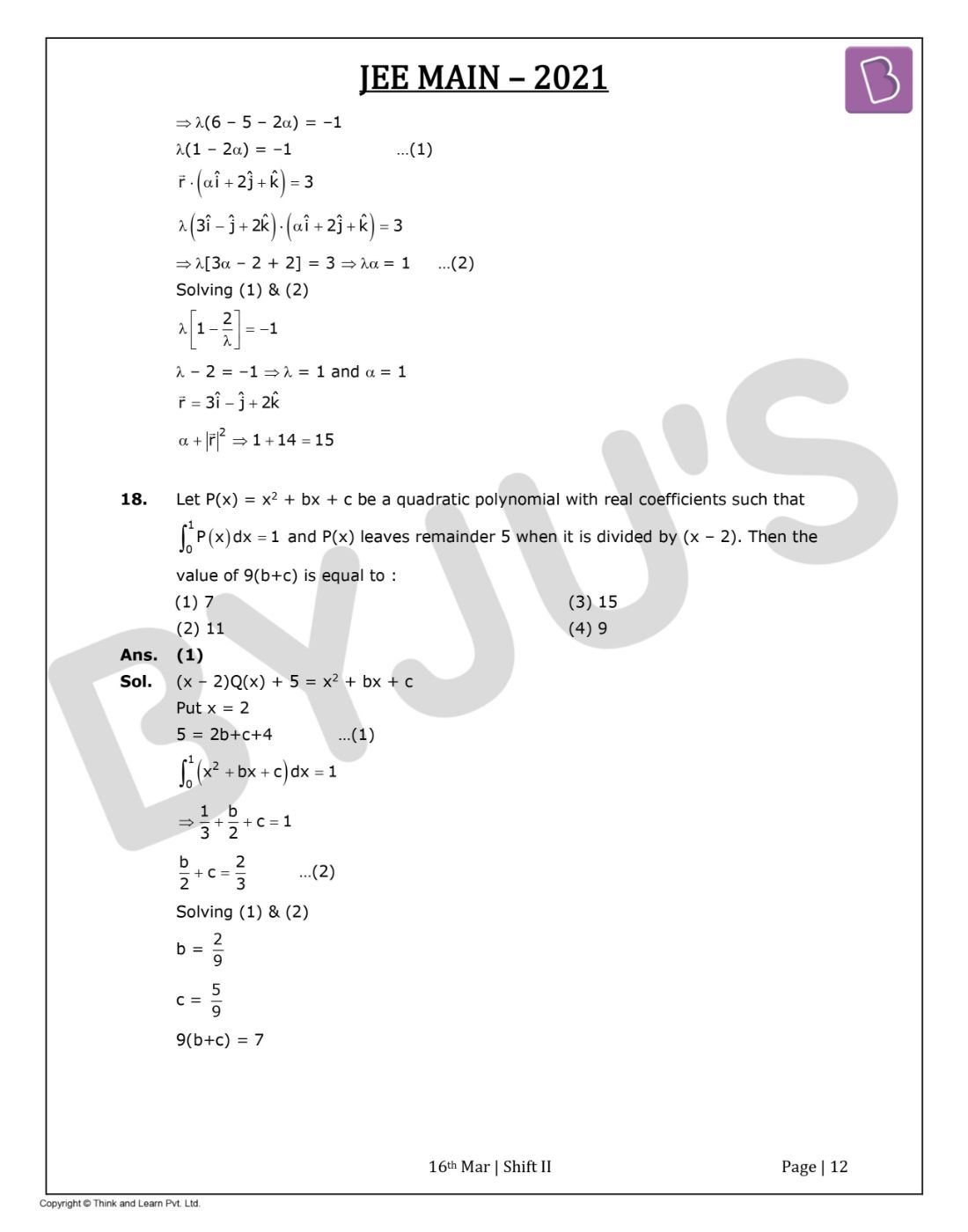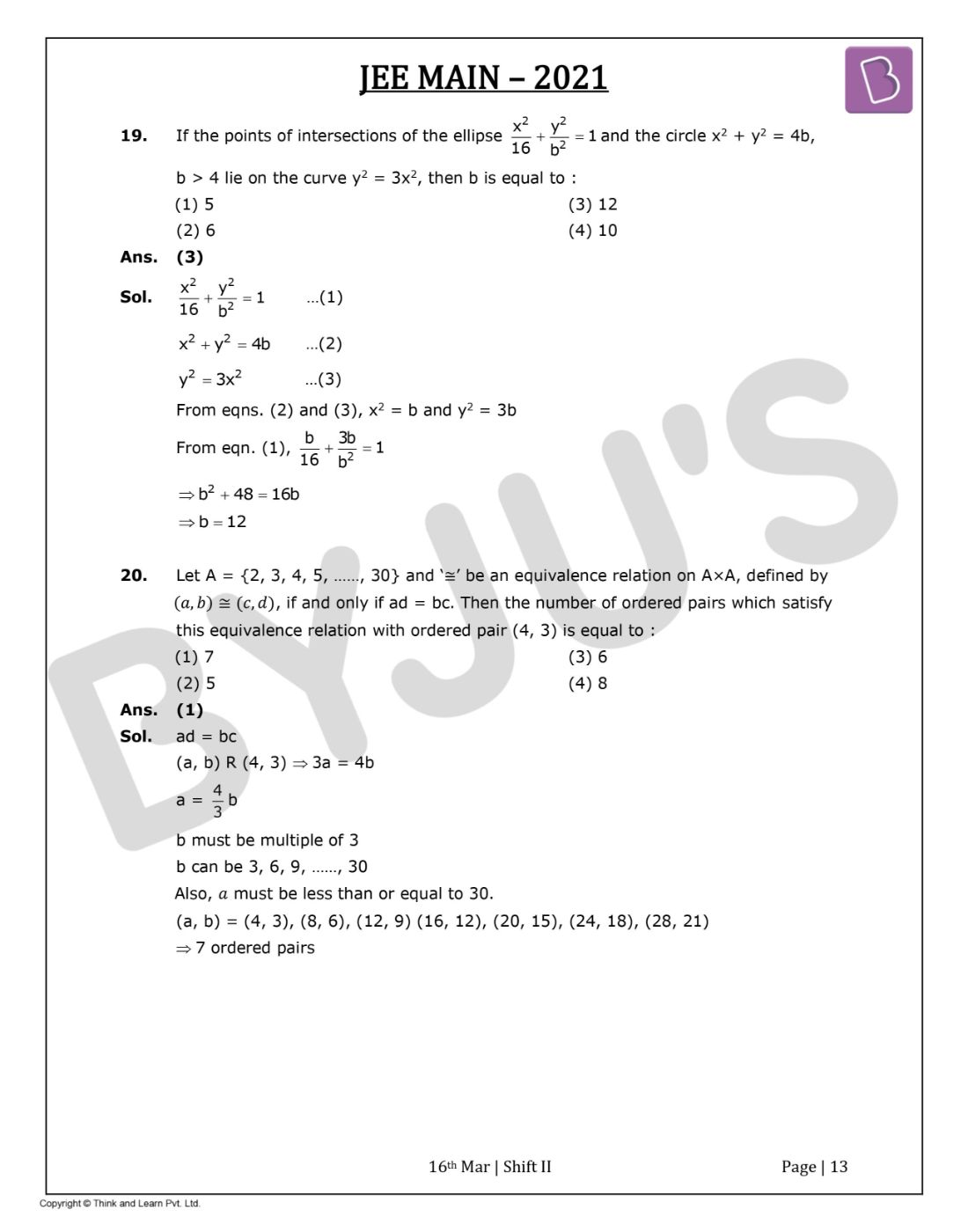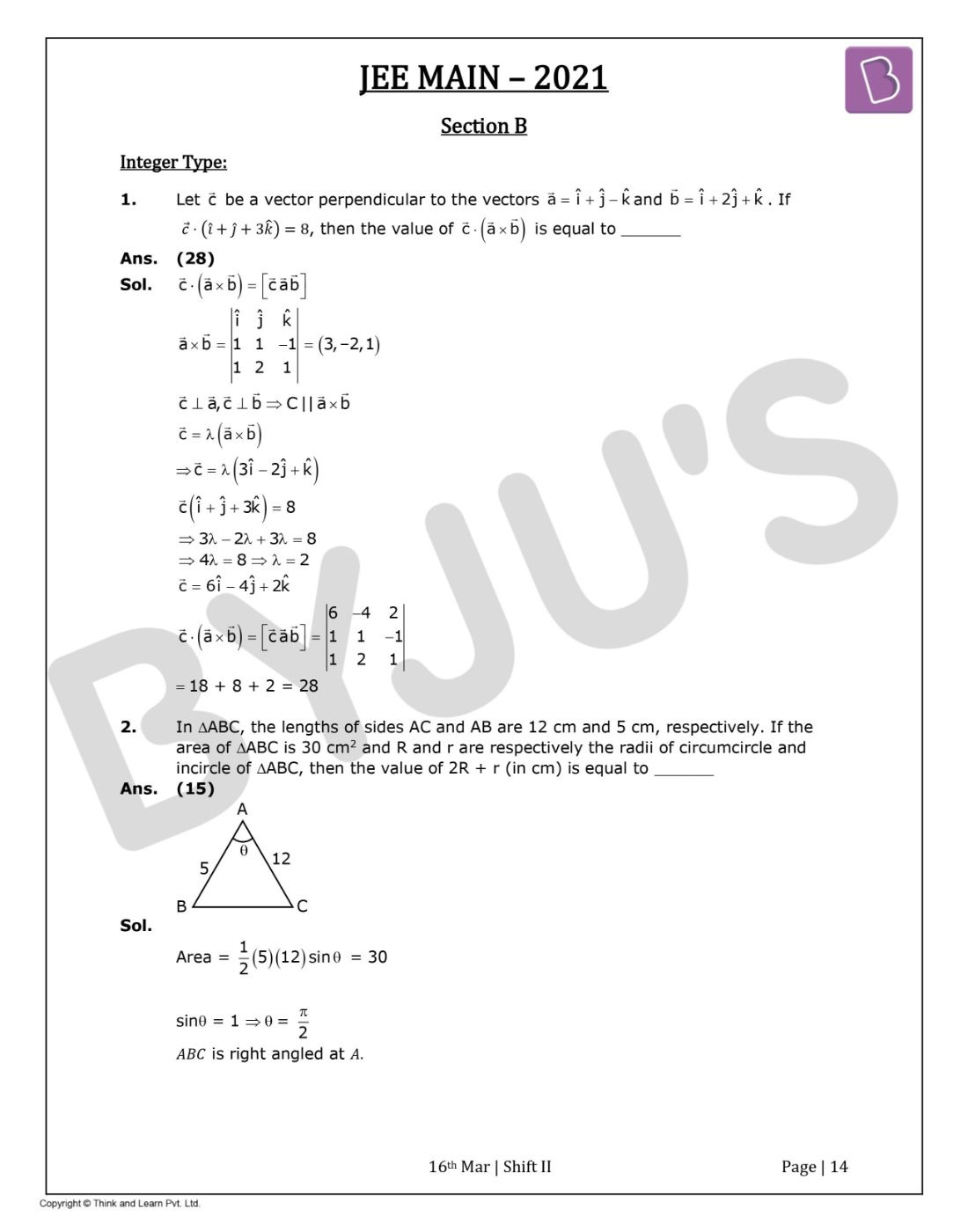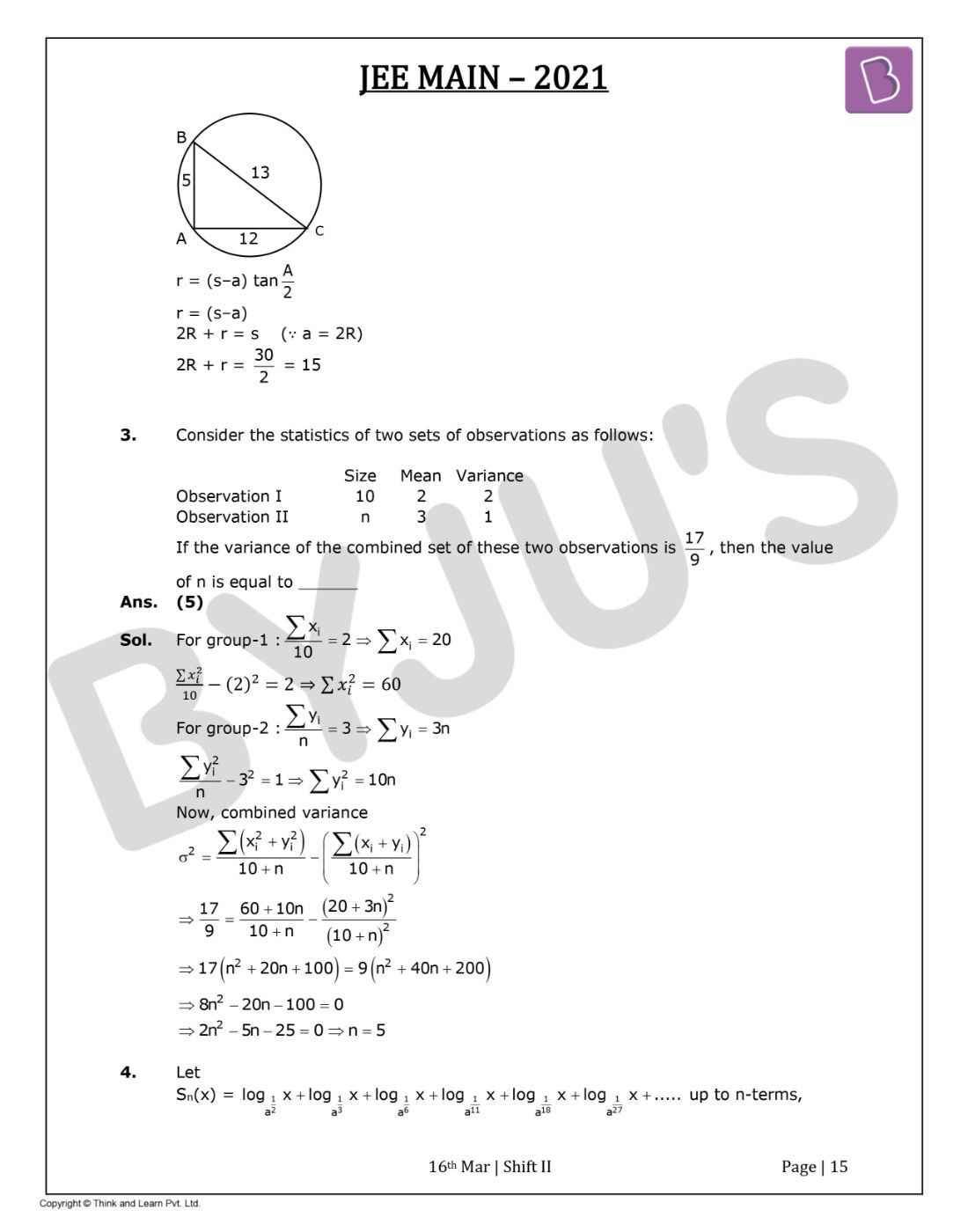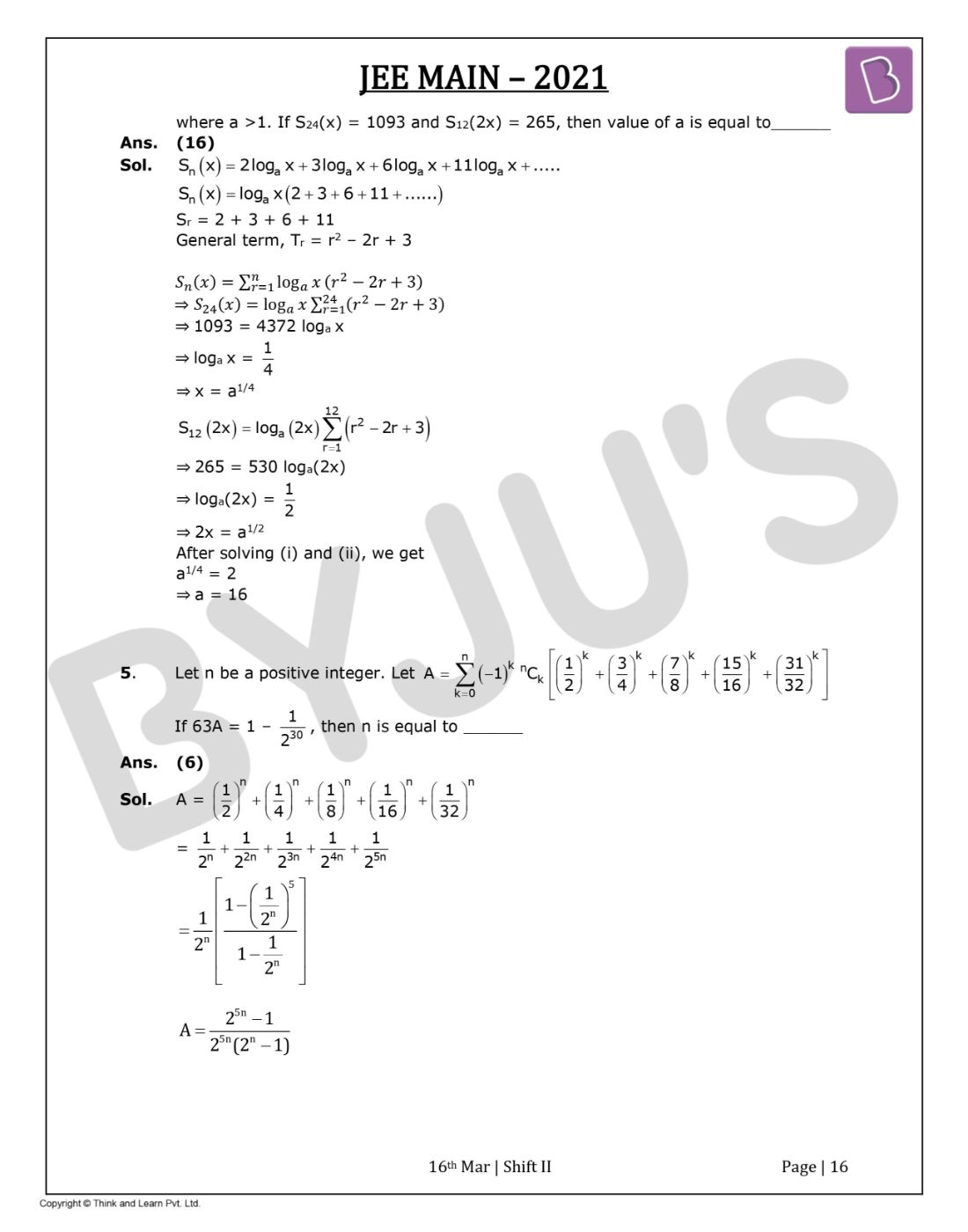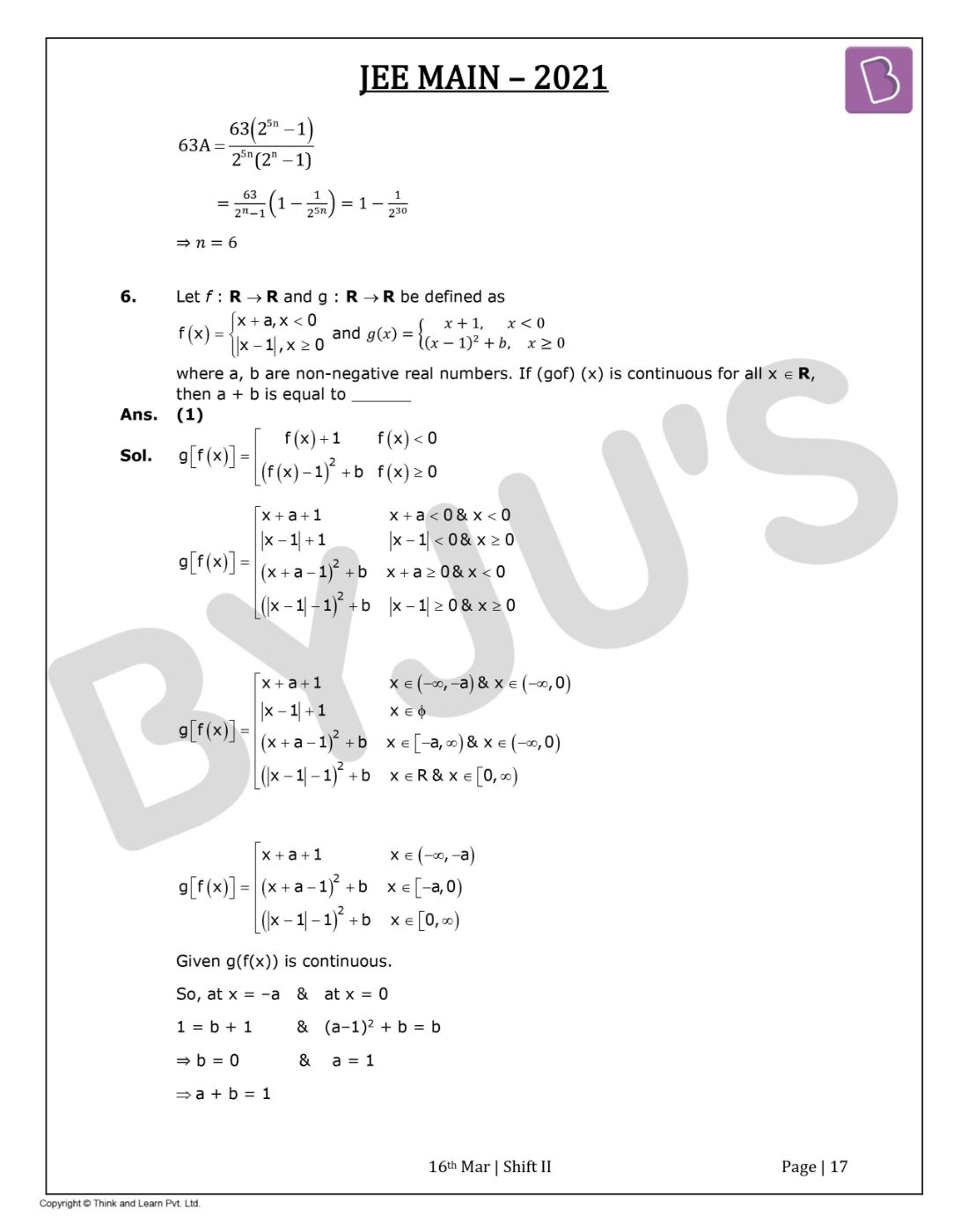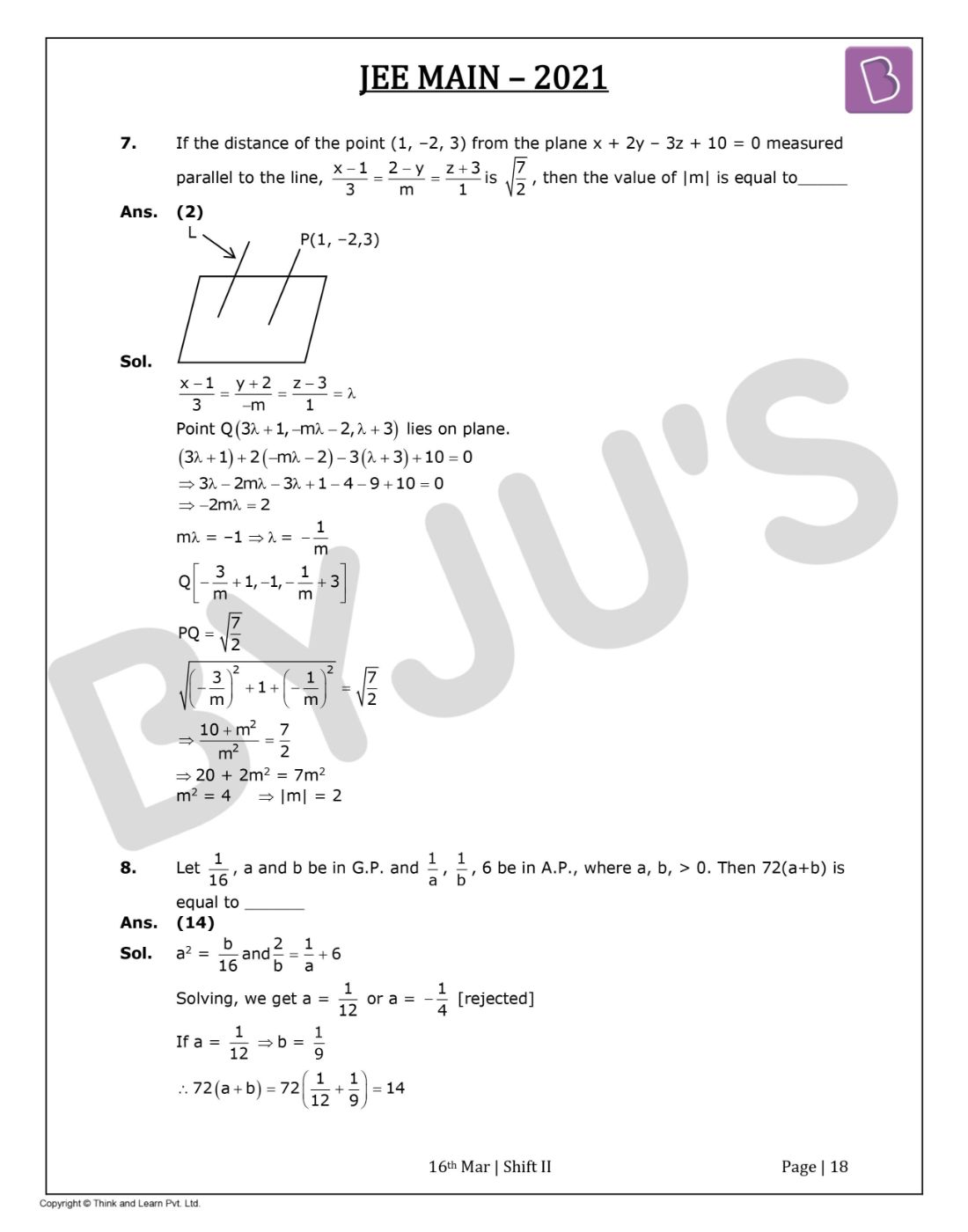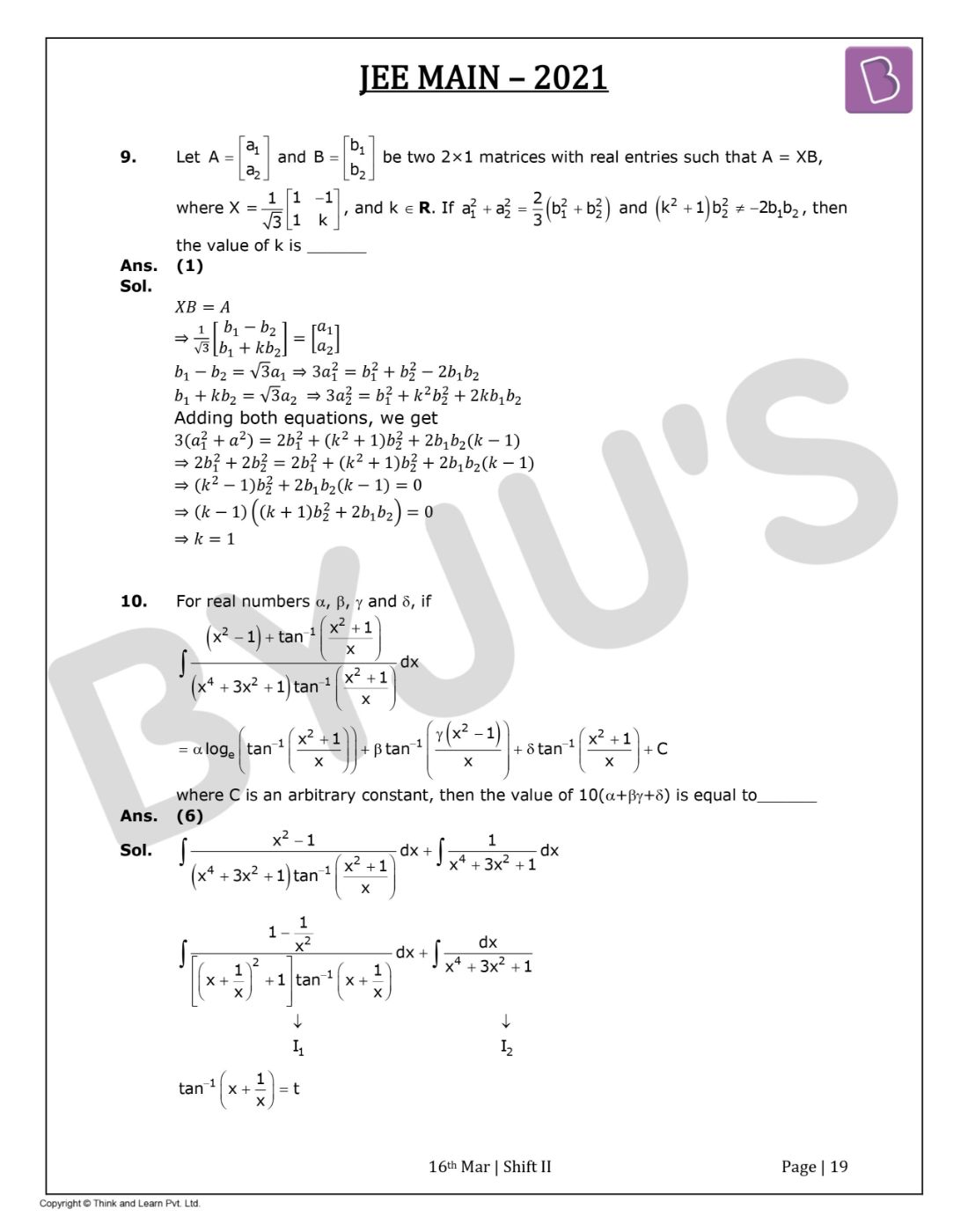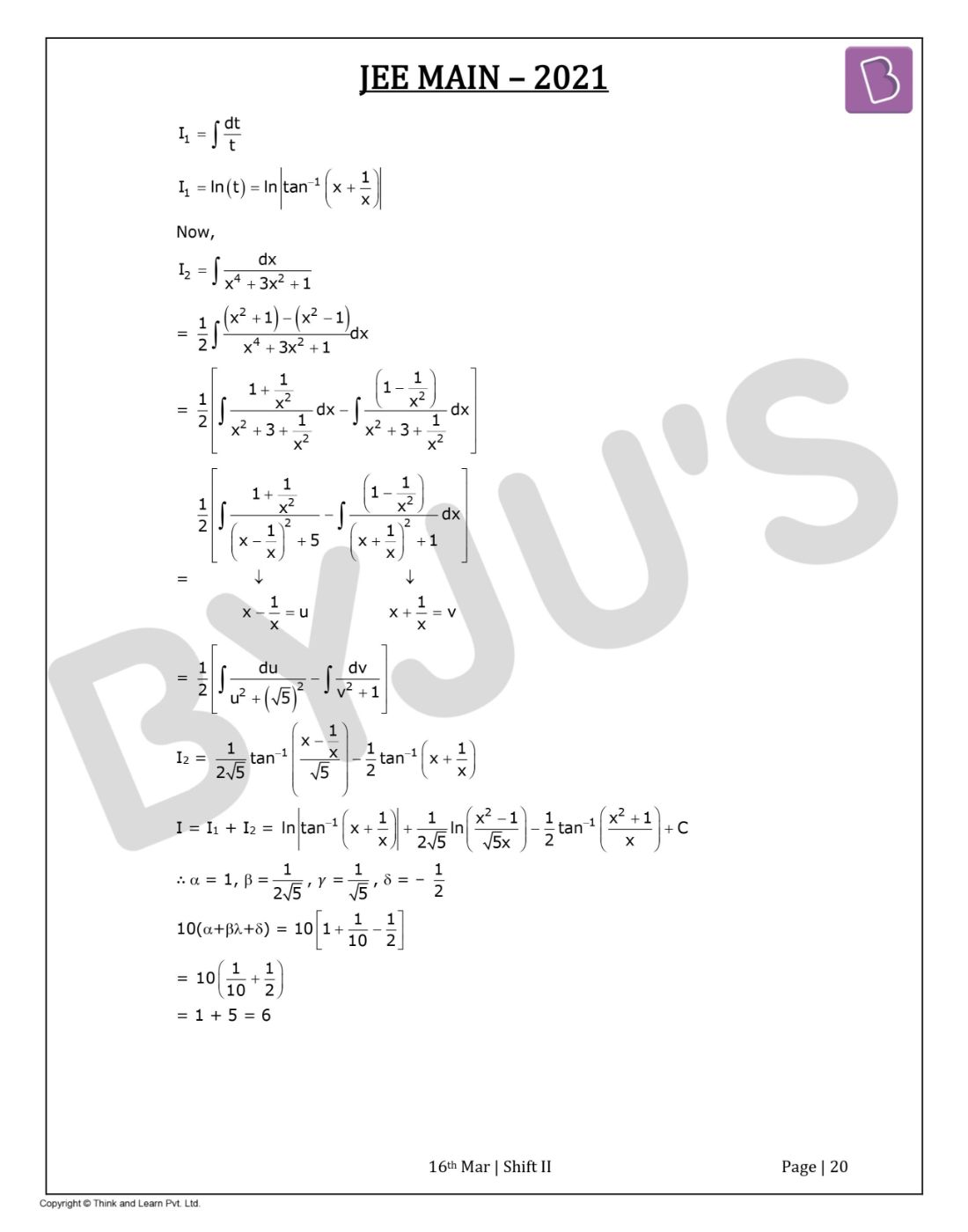Win up to 100% scholarship on Aakash BYJU'S JEE/NEET courses with ABNAT Win up to 100% scholarship on Aakash BYJU'S JEE/NEET courses with ABNAT

# JEE Main 2021 March 16 – Shift 2 Maths Question Paper with Solutions

JEE Main 2021 March 16th Shift 2 Maths Question Paper is provided here along with solutions that have been prepared by our subject experts. Students can go through the solutions or download the solved paper in a PDF format for offline practice to improve their problem solving skills. While referring to these JEE Main 2021 Maths questions and solutions students will also be able to learn about the different types of problems asked in the entrance exam and at the same time develop better speed and acccuracy to solve them.

JEE Main 2021 March 16th Shift 2 Maths Question Paper

Section A

Question 1: The least value of |z| where z is a complex number which satisfies the inequality exp [{(|z| + 3) (|z| – 1)} / {||z| + 1}] loge 2 ≥ log√2 |5 √7 + 9i|, i = √-1, is equal to:

a. 2
b. 3
c. 8
d. √5

2[{(|z| + 3) (|z| – 1)} / {|z| + 1}] ≥ 23 ⇒ [{(|z| + 3) (|z| – 1)} / {||z| + 1}] ≥ 3

|z|2 + 2 |z| – 3 ≥ 3 |z| + 3

|z|2 – |z| – 6 ≥ 0

(|z| – 3) (|z| + 2) ≥ 0

|z|min = 3

Question 2: Let f : S → S where S = (0, ∞) be a twice differentiable function such that f (x + 1) = x f (x). If g : S → R be defined as g (x) = loge f (x), then the value of |g”(5) – g”(1)| is equal to:

a. 197 / 144
b. 187 / 144
c. 205 / 144
d. 1

f(x+1) = xf(x)

g(x+1) = loge(f(x+1))

g(x+1) = loge x + loge f(x)

g(x+1) – g(x) = loge x

g’’(x+1) – g’’(x) = – 1 / x2

g’’(2) – g’’(1) = –1

g’’(3) – g’’(2) = – 1 / 4

g’’(4) – g’’(3) = – 1 / 9

g’’(5) – g’’(4) = – 1 / 16

g’’(5) – g’’(1) =- [1 + (1 / 4) + (1 / 9) + (1 / 16)]

|g’’(5) – g’’(1)| = [144 + 36 + 16 + 9] / [16 * 9] = 205 / 144

Question 3: If y = y (x) is the solution of the differential equation (dy / dx) + (tan x) y = sinx, 0 ≤ x ≤ π / 3, with y(0) = 0, then y (π / 4) equal to:

a. loge 2
b. (1 / 2) loge 2
c. (1 / 2 √2) loge 2
d. (1 / 4) loge 2

I.F. = e∫tanx dx

= eln secx

= sec x

Solution of the equation:

y(sec x) = ∫sin x sec x dx

y / (cosx) = ln (secx) + c

Put x = 0, c = 0

∴ y = cos x ln(sec x)

Put x = π / 4

y = (1 / √2) ln √2 = (1 / 2√2) ln 2

y = (ln 2) / (2√2)

Question 4: If the foot of the perpendicular from point (4, 3, 8) on the line L1 : [x – a] / l = [y – 2] / 3 = [z – b] / 4, l ≠ 0 is (3, 5, 7), then the shortest distance between the line L1 and line L2 : [x – 2] / 3 = [y – 4] / 4 = [z – 5] / 5 is equal to:

a. √2 / 3
b. 1 / √3
c. 1 / 2
d. 1 / √6

(3, 5, 7) lies on given line L1

[3 – a] / l = 3 / 3 = [7 – b] / 4

[7 – b] / 4 = 1 ⇒ b = 3

[3 – a] / l = 1 ⇒ 3 – a = l

A (4, 3, 8)

B (3, 5, 7)

DR’s of AB = (1, –2, 1)

AB ⊥ line L1

(1)(l) + (–2)(3) + 4(1) = 0

⇒ l = 2

a = 1

a = 1, b = 3, l = 2

[x – 1] / 2 = [y – 2] / 3 = [z – 3] / 4

[x – 2] / 3 = [y – 4] / 4 = [z – 5] / 5

SD =

$$\begin{array}{l}\frac{\begin{vmatrix} 1 &2 &2 \\ 2&3 &4 \\ 3&4 &5 \end{vmatrix}}{\begin{Vmatrix} i &j &k \\ 2&3 &4 \\ 3&4 &5 \end{Vmatrix}}\end{array}$$
= 1 / √6

Question 5: If (x, y, z) be an arbitrary point lying on a plane P which passes through the points (42, 0, 0), (0, 42, 0) and (0, 0, 42), then the value of the expression 3 + [x – 11] / [(y – 19)2 (z – 12)2] + [y – 19] / [(x – 11)2 (z – 12)2] + [z – 12] / [(z – 11)2 (y – 19)2] – [x + y + z] / [14 (x – 11) (y – 19) (z – 12)] is equal to :

a. 3
b. 0
c. 39
d. – 45

Equation of plane is x + y + z = 42 or, (x − 11)+ (y − 19)+ (z −12) = 0.

Now, [x – 11] / [(y – 19)2 (z – 12)2] + [y – 19] / [(x – 11)2 (z – 12)2] + [z – 12] / [(z – 11)2 (y – 19)2]

= [(x – 11)3 + (y – 19)3 + (z – 12)3] / [(x – 11)2 (y – 19)2 (z – 12)2]

= [3 (x – 11) (y – 19) (z – 12)] / [(x – 11)2 (y – 19)2 (z – 12)2] [If a + b + c = 0, then a3 + b3 + c3 = 3abc]

= 3 / [(x – 11) (y – 19) (z – 12)]

The given expression is equal to 3 + 3 / [(x – 11) (y – 19) (z – 12)] – 3 / [(x – 11) (y – 19) (z – 12)] = 3

Question 6: Consider the integral I = ∫010 {[x] e[x]} / [ex-1] dx, where [x] denotes the greatest integer less than or equal to x. Then the value of I is equal to :

a. 45 (e – 1)
b. 45 (e + 1)
c. 9(e – 1)
d. 9(e + 1)

I = ∫010 {[x] e[x]} / [ex-1] dx

= ∫010 [x] e[x]+1-xdx

= ∫12 e2-x dx + ∫23 2 e3-x dx + ∫34 3 e4-x dx + ……… ∫910 9 e10-x dx

= – {(1 – e) + 2(1 – e) + 3(1 – e) +……+ 9(1 – e)}

= 45(e – 1)

Question 7: Let A (–1, 1), B (3, 4) and C(2, 0) be given three points. A line y = mx, m > 0, intersects lines AC and BC at point P and Q respectively. Let A1 and A2 be the areas of ΔABC and ΔPQC respectively, such that A1 = 3A2, then the value of m is equal to:

a. 4 / 15
b. 1
c. 2
d. 3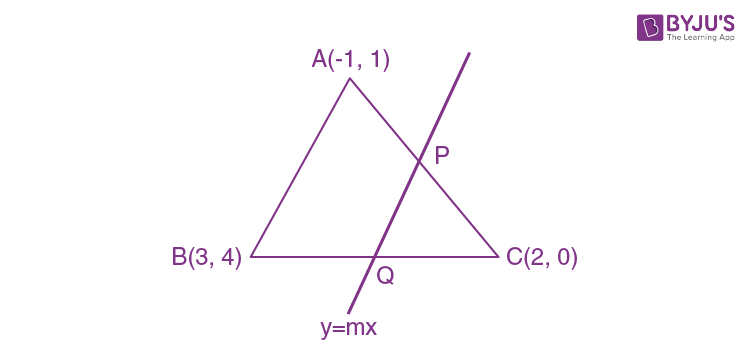A1 = Area of ΔABC =

$$\begin{array}{l}\frac{1}{2}\begin{Vmatrix} -1 &1 &1 \\ 2&0 &1 \\ 3&4 &1 \end{Vmatrix}\end{array}$$

A1 = 13 / 2

Equation of line AC is y – 1 = (- 1 / 3) (x + 1)

Solving it with line y = mx, we get P [2 / (3m + 1), 2m / (3m + 1)]

Equation of line BC is y – 0 = 4(x – 2)

Solving it with line y = mx, we get Q [- 8 / (m – 4), – 8m / (m – 4)]

A2 = Area of ΔPQC =

$$\begin{array}{l}\frac{1}{2}\begin{Vmatrix} 2 &0 &1 \\ 2/(3m+1)&2m/(3m+1) &1 \\ -8/(m-4)&-8m/(m-4) &1 \end{Vmatrix}\end{array}$$
= A1 / 3 = 13 / 6

[26m2] / [3m2 – 11m – 4] = ± 13 / 6

⇒ 12m2 = ± (3m2 – 11m – 4)

Taking +ve sign,

9m2 + 11m + 4 = 0 (Rejected ∵ m is imaginary)

Taking –ve sign,

15m2 – 11m – 4 = 0

m = 1, – 4 / 15

m = 1 as m > 0

Question 8: Let f be a real-valued function, defined on R – {–1, 1} and given by f(x) = 3 loge |(x – 1) / (x + 1)| – 2 / (x – 1). Then in which of the following intervals, function f (x) is increasing?

a. (- ∞, – 1) ⋃ ([1 / 2, ∞) – {1})
b. (- 1, 1 / 2]
c. (–∞, ∞) – {–1, 1}
d. (- ∞, 1 / 2] – {- 1}

f’ (x) = [(x + 1) / (x – 1) * (x + 1 – (x – 1)) / (x + 1)2] 3 + 2 / (x – 1)2 = 6 / (x – 1) (x + 1) + 2 / (x – 1)2

= [4(2x – 1)]/[(x + 1)(x – 1)2]

x ∈ (- ∞, – 1) ⋃ ([1 / 2, ∞) – {1})

Question 9: Let the lengths of intercepts on x-axis and y-axis made by the circle x2 + y2 + ax + 2ay + c = 0, (a < 0) be 2 √2 and 2 √5, respectively. Then the shortest distance from origin to a tangent to this circle which is perpendicular to the line x + 2y = 0, is equal to:

a. √10
b. √6
c. √11
d. √7

2 √(a2 / 4 – c) = 2√2

√(a2 – 4c) = 2√2

a2 – 4c = 8 — (1)

2√(a2 – c) = 2√5

a2 – c = 5 — (2)

(2) – (1), we get

3c = –3 ⇒ c = –1

a2 = 4 ⇒ a = –2

x2 + y2 – 2x – 4y – 1 = 0

Equation of tangent 2x – y + λ = 0

∴ p = r

|(0 – 0 + λ) / √5| = √6

λ = ± √30

∴ Tangents are 2x – y ± √30 = 0

Distance from origin = √30 / √5 = √6

Question 10: Let A denote the event that a 6-digit integer formed by 0, 1, 2, 3, 4, 5, 6 without repetitions, be divisible by 3. Then the probability of event A is equal to:

a. 4 / 9
b. 9 / 56
c. 3 / 7
d. 11 / 27

Total case = 6∟6

Fav. case = (0, 1, 2, 3, 4, 5) + (0, 1, 2, 4, 5, 6) + (1, 2, 3, 4, 5, 6)

= 5 ∟5 + 5 ∟5 + ∟6

= 1920

Probability = 1920 / 6∟6 = 4 / 9

Question 11: Let α ∈ R be such that the function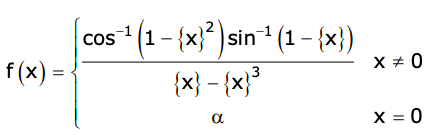is continuous at x = 0, where {x} = x – [x], [x] is the greatest integer less than or equal to x. Then:

a. α = π / 4
b. No such α exists
c. α = 0
d. α = π / √2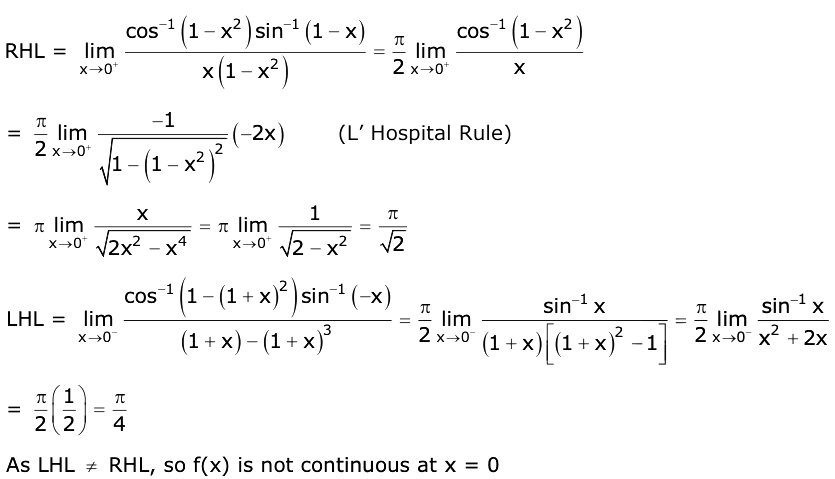Question 12: The maximum value of f (x) =

$$\begin{array}{l}\begin{vmatrix} sin^2x &1+cos^2x &cos2x \\ 1+sin^2x&cos^2x &cos2x \\ sin^2x&cos^2x &sin2x \end{vmatrix}\end{array}$$
, x ∈ R is:

a. √7
b. √5
c. 5
d. 3 / 4

C1 → C1 + C2

$$\begin{array}{l}\begin{vmatrix} 2 &1+cos^2x &cos2x \\ 2&cos^2x &cos2x \\ 1&cos^2x &sin2x \end{vmatrix}\end{array}$$

R1 → R1 – R2

$$\begin{array}{l}\begin{vmatrix} 0 &1 &0 \\ 2&cos^2x &cos2x \\ 1&cos^2x &sin2x \end{vmatrix}\end{array}$$

= (–1)[2sin2x – cos2x] = cos2x – 2sin2x

Maximum value = √5

Question 13: Consider a rectangle ABCD having 5, 7, 6, 9 points in the interior of the line segments AB, CD, BC, DA respectively. Let α be the number of triangles having these points from different sides as vertices and β be the number of quadrilaterals having these points from different sides as vertices. Then (β – α) is equal to:

a. 1890
b. 795
c. 717
d. 1173

α = 6C1 7C1 9C1 + 5C1 7C1 9C1 + 5C1 6C1 9C1 + 5C1 6C1 7C1

= 378 + 315 + 270 + 210

= 1173

β = 5C1 6C1 7C1 9C1 = 1890

⇒ β – α = 1890 – 1173 = 717

Question 14: Let C be the locus of the mirror image of a point on the parabola y2 = 4x with respect to the line y = x. Then the equation of tangent to C at P(2, 1) is:

a. 2x + y = 5
b. x + 2y = 4
c. x + 3y = 5
d. x – y = 1Image of y2 = 4x w.r.t. y = x is x2 = 4y

Tangent from (2, 1)

xx1 = 2(y + y1)

2x = 2(y + 1)

x = y + 1

Question 15: Given that the inverse trigonometric functions take principal values only. Then, the number of real values of x which satisfy sin-1 (3x / 5) + sin-1 (4x / 5) = sin-1 x =

a. 1
b. 2
c. 3
d. 0

Taking sin on both sides, (3x / 5) √[1 – (16x2 / 25)] + (4x / 5) √[1 – (9x2 / 25)] = x

3x √(25 – 16x2) = 25x – 4x √(25 – 9x2)

x = 0 or 3√(25 – 16x2) = 25 – 4√(25 – 9x2)

9 (25 – 16x2) = 625 – 200 √(25 – 9x2) + 16 (25 – 9x2)

200 √(25 – 9x2) = 800

√(25 – 9x2) = 4

x2 = 1

x = ± 1

Total number of solutions = 3

Question 16: Let C1 be the curve obtained by the solution of the differential equation 2xy (dy / dx) = y2 – x2, x > 0. Let curve C2 be the solution of 2xy / (x2 – y2) = dy / dx. If both the curves pass through (1, 1), then the area enclosed by the curves C1 and C2 is equal to :

a. (π / 2) – 1
b. (π / 4) + 1
c. π – 1
d. π + 1

dy / dx = [y2 – x2] / 2xy

Put y = vx

v + x (dv / dx) = [v2x2 – x2] / 2vx2 = [v2 – 1] / 2v

x (dv / dx) = [v2 – 1 – 2v2] / 2v = – (v2 + 1) / 2v

2v / (v2 + 1) dv = – dx / x

ln (v2 + 1) = – ln x + ln c ⇒ v2 + 1 = c / x

(y2 / x2) + 1 = c / x ⇒ x2 + y2 = cx

It passes through (1, 1)

∴ x2 + y2 – 2x=0

Similarly, for second differential equation dy / dx = [x2 – y2] / 2xy

equation of curve is x2 + y2 – 2y = 0

Now the required area is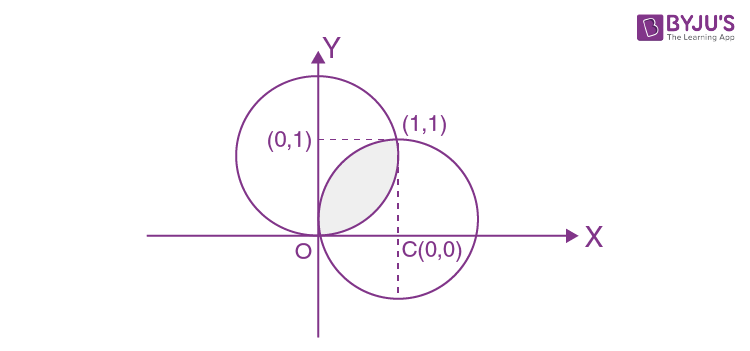= [(1 / 4) * π * 12 – (1 / 2) (1) (1)] * 2

= [(π / 2) – 1] square units

Question 17: Let a = i + 2j – 3k and b = 2i – 3j + 5k. If r x a = b x r, r . (ɑi + 2j + k) = 3 and r . (2i + 5j – ɑk) = – 1, ɑ ∈ R, then the value of ɑ + |r|2 =

a. 11
b. 15
c. 9
d. 13

r x a = – r x b

r x (a + b) = 0 (a + b = 3i – j + 2k)

r || (a + b)

r = λ (a + b)

∵ r . (2i + 5j – ɑk) = – 1

λ [3i – j + 2k] . [2i + 5j – ɑk] = – 1

⇒ λ(6 – 5 – 2α) = –1

λ(1 – 2α) = –1 …(1)

r (ɑi + 2j + k) = 3

λ (3i – j + 2k) . (ɑi + 2j + k) = 3

⇒ λ[3α – 2 + 2] = 3 ⇒ λα = 1 …(2)

Solving (1) & (2)

λ [1 – (2 / λ)] = – 1

λ – 2 = –1 ⇒ λ = 1 and α = 1

r = 3i – j + 2k

α + |r|2 = 1 + 14 = 15

Question 18: Let P(x) = x2 + bx + c be a quadratic polynomial with real coefficients such that ∫01 P (x) dx and P(x) leaves remainder 5 when it is divided by (x – 2). Then the value of 9 (b+c) is equal to :

a. 7
b. 11
c. 15
d. 9

(x – 2) Q (x) + 5 = x2 + bx + c

Put x = 2

5 = 2b + c + 4 …(1)

01 (x2 + bx + c) dx = 1

(1 / 3) + (b / 2) + c = 1

(b / 2) + c = 2 / 3 …(2)

Solving (1) & (2)

b = 2 / 9

c = 5 / 9

9 (b + c) = 7

Question 19: If the points of intersections of the ellipse (x2 / 16) + (y2 / b2) = 1 and the circle x2 + y2 = 4b, b > 4 lie on the curve y2 = 3x2, then b is equal to :

a. 5
b. 6
c. 12
d. 10

(x2 / 16) + (y2 / b2) = 1 …(1)

x2 + y2 = 4b …(2)

y2 = 3x2 …(3)

From eqns. (2) and (3), x2 = b and y2 = 3b

From eqn. (1), (b / 16) + (3b / b2 ) = 1

b2 + 48 = 16b

b = 12

Question 20: Let A = {2, 3, 4, 5, ……, 30} and ‘⩭’ be an equivalence relation on A×A, defined by (a, b) ⩭ (c, d), if and only if ad = bc. Then the number of ordered pairs which satisfy this equivalence relation with ordered pair (4, 3) is equal to :

a. 7
b. 5
c. 6
d. 8

a = bc

(a, b) R (4, 3) ⇒ 3a = 4b

a = (4 / 3) b

b must be multiple of 3

b can be 3, 6, 9, ……, 30

Also, a must be less than or equal to 30.

(a, b) = (4, 3), (8, 6), (12, 9) (16, 12), (20, 15), (24, 18), (28, 21)

⇒ 7 ordered pairs

Section B

Question 21: Let

$$\begin{array}{l}\vec{c}\end{array}$$
be a vector perpendicular to the vectors a = i + j – k and b = i + 2j + k. If c . (i + j + 3k) = 8, then the value of c . (a x b) is equal to ______

$$\begin{array}{l}\vec{c}.(\vec{a}\times\vec{b})=[\vec{c}\ \vec{a}\ \vec{b}]\\ (\vec{a}\times\vec{b})= \begin{vmatrix} i &j &k \\ 1&1 &-1 \\ 1&2 &1 \end{vmatrix}=(3,-2,1)\\ \vec{c}\perp \vec{a},\vec{c}\perp \vec{b}\Rightarrow C||(\vec{a}\times\vec{b})\\ \vec{c}=\lambda (\vec{a}\times\vec{b})\\ \vec{c}=\lambda (3i-2j+k)\\ \vec{c}(i+j+3k)=8\\ 3\lambda-2\lambda+3\lambda=8\\ \lambda=2\\ \vec{c}=6i-4j+2k\\ \vec{c}.(\vec{a}\times\vec{b})=[\vec{c}\ \vec{a}\ \vec{b}]=\begin{vmatrix} 6 &-4 &2 \\ 1&1 &-1 \\ 1&2 &1 \end{vmatrix}\\ =18+8+2=28\end{array}$$

Question 22: In ΔABC, the lengths of sides AC and AB are 12 cm and 5 cm, respectively. If the area of ΔABC is 30 cm2 and R and r are respectively the radii of circumcircle and incircle of ΔABC, then the value of 2R + r (in cm) is equal to ______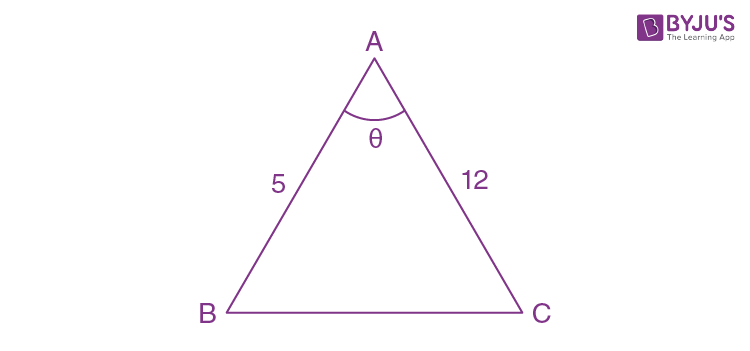Area = (1 / 2) * 5 * 12 * sin θ = 30

sin θ = 1 ⇒ θ = π / 2

ABC is right-angled at A.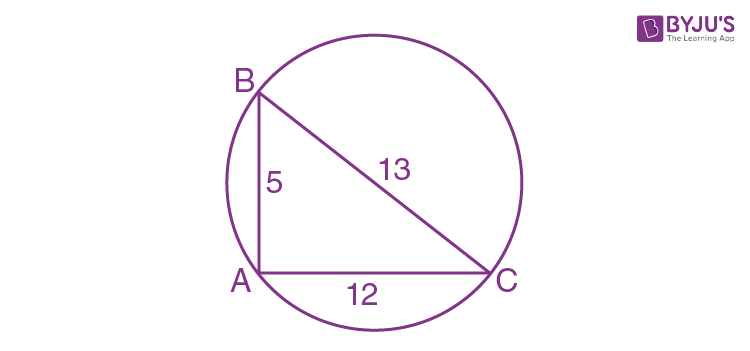r = (s – a) tan (A / 2)

r = (s – a)

2R + r = s ( a = 2R)

2R + r = 30 / 2 = 15

Question 23: Consider the statistics of two sets of observations as follows:

 Size Mean Variance Observation I 10 2 2 Observation II n 3 1

If the variance of the combined set of these two observations is 17 / 9, then the value of n is equal to ______

For group-1: ∑xi / 10 = 2 ⇒ ∑xi = 20

∑xi2 / 10 – (22) = 2

∑xi2 = 60

For group-2 : ∑yi / n = 3

∑yi = 3n

∑yi2 / n – 32 = 1

∑yi2 = 10n

Now, combined variance

𝜎2 = [∑(xi2 + yi2) / (10 + n)] – [∑ (xi + yi) / (10 + n)]2

(17 / 9) = [60 + 10n] / [10 + n] – [20 + 3n]2 / [10 + n]2

17 (n2 + 20n + 100) = 9 (n2 + 40n + 200)

8n2 – 20n – 100 = 0

2n2 – 5n – 25 = 0

n = 5

Question 24: Let Sn(x) =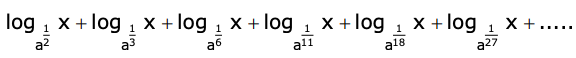up to n-terms, where a >1. If S24(x) = 1093 and S12(2x) = 265, then value of a is equal to______

Sn (x) = 2 loga x + 3 loga x + 6 loga x + 11 loga x + ……..

Sn (x) = loga x (2 + 3 + 6 + 11 + …… )

Sr = 2 + 3 + 6 + 11

General term, Tr = r2 – 2r + 3

Sn (x) = ∑r=1n x (r2 – 2r + 3)

S24 (x) = x ∑r=124 (r2 – 2r + 3)

1093 = 4372 loga x

loga x = 1 / 4

x = a1/4

S12 (2x) = loga (2x) ∑r=112 (r2 – 2r + 3)

265 = 530 loga(2x)

loga(2x) = 1 / 2

2x = a1/2

After solving (i) and (ii), we get

a1/4 = 2

a = 16

Question 25: Let n be a positive integer. Let A = ∑k=0n (-1)knCk [(1 / 2)k + (3 / 4)k + (7 / 8)k + (15 / 16)k + (31 / 32)k]. If 63A = 1 – (1 / 230), then n is equal to ______

A = (1 / 2)n + (1 / 4)n + (1 / 8)n + (1 / 16)n + (1 / 32)n

= (1 / 2n) + (1 / 22n) + (1 / 23n) + (1 / 24n) + (1 / 25n)

= (1 / 2n) [1 – (1 / 2n)]5 / [1 – (1 / 2n)]

A = [25n – 1] / [25n (2n – 1)]

63A = [63 (25n – 1)] / [25n (2n – 1)]

= (63 / (2n – 1)) [1 – (1 / 25n)] = 1 – (1 / 230)

n = 6

Question 26: Let f : R → R and g : R → R be defined as f (x) =

$$\begin{array}{l}\left\{\begin{matrix} x+a, &x<0 \\ |x-1|, &x\geq 0 \end{matrix}\right.\end{array}$$
and g (x) =
$$\begin{array}{l}\left\{\begin{matrix} x+1, &x<0 \\ (x-1)^2+b, &x\geq 0 \end{matrix}\right.\end{array}$$
where a, b are non-negative real numbers. If (gof) (x) is continuous for all x ∈ R, then a + b is equal to ______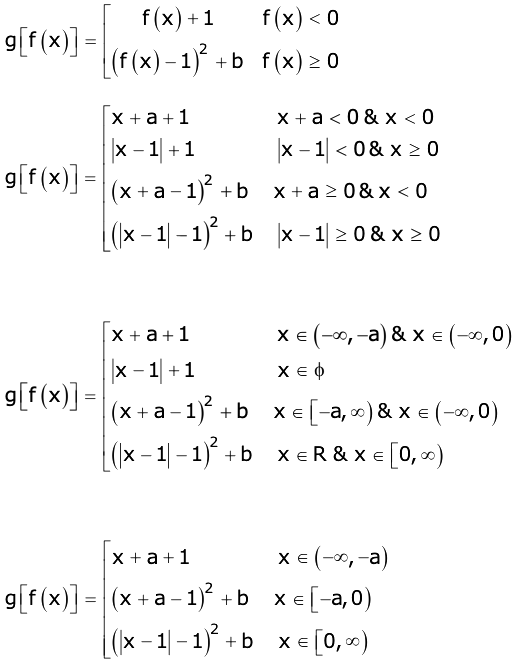Given g(f(x)) is continuous.

So, at x = –a & at x = 0

1 = b + 1 & (a–1)2 + b = b

b = 0 & a = 1

⇒ a + b = 1

Question 27: If the distance of the point (1, – 2, 3) from the plane x + 2y – 3z + 10 = 0 measured parallel to the line, [x – 1] / 3 = [2 – y] / m = [z + 3] / 1 is √7 / 2, then the value of |m| is equal to_____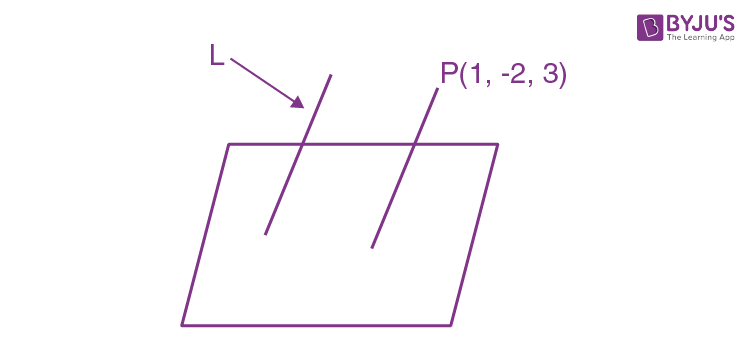[x – 1] / 3 = [y + 2] / – m = [z – 3] / 1 = λ

Point Q (3λ + 1, – mλ – 2, λ + 3) lies on the plane.

(3λ + 1) + 2 (- mλ – 2) – 3 (λ + 3) + 10 =0

3λ – 2mλ – 3λ + 1 – 4 – 9 + 10 = 0

– 2mλ = 2

mλ = –1 ⇒ λ = – 1 / m

Q [(- 3 / m) + 1, – 1, (- 1 / m) + 3]

PQ = √(7/2)

√(- 3 / m)2 + 1 + (- 1 / m)2 = √(7/2)

[10 + m2] / m2 = 7 / 2

⇒ 20 + 2m2 = 7m2

m2 = 4 ⇒ |m| = 2

Question 28: Let 1 / 16, a and b be in G.P. and 1/a, 1/b, 6 be in A.P., where a, b, > 0. Then 72 (a + b) is equal to ______

a2 = b / 16 and 2 / b = (1 / a) + 6

Solving, we get a = 1 / 12 or a = – 1 / 4 [rejected]

If a = 1 / 12 ⇒ b = 1 / 9

72 (a + b) = 72 [(1 / 12) + (1 / 9)] = 14

Question 29: Let

$$\begin{array}{l}A=\begin{bmatrix} a_{1}\\ a_{2} \end{bmatrix}\end{array}$$
and
$$\begin{array}{l}B=\begin{bmatrix} b_{1}\\ b_{2} \end{bmatrix}\end{array}$$
be two 2 × 1 matrices with real entries such that A = XB, where X =
$$\begin{array}{l}\frac{1}{\sqrt3}\begin{bmatrix} 1 &-1 \\ 1&k \end{bmatrix}\end{array}$$
, and k ∈ R. If (a12 + a22) = (2 / 3) (b12 + b22) and (k2 + 1) b22 ≠ – 2b1b2, then the value of k is ______

XB = A

(1 / √3)

$$\begin{array}{l}\begin{bmatrix} b_{1}-b_{2}\\ b_{1}+kb_{2} \end{bmatrix}=\begin{bmatrix} a_{1}\\ a_{2} \end{bmatrix}\end{array}$$

b1 – b2 = √3a1

3a12 = b12 + b22 – 2b1b2

b1 + kb2 = √3a2

3a22 = b12 + k2b22 + 2kb1b2

Adding both equations, 3 (a12 + a2) = 2b12 + (k2 + 1) b22 + 2b1b2 (k – 1)

(k2 – 1) b22 + 2b1b2 (k – 1) = 0

(k – 1) [(k + 1) b22 + 2b1b2 = 0

k = 1

Question 30: For real numbers α, β, γ and δ, if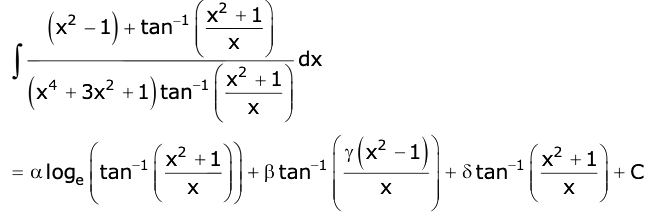where C is an arbitrary constant, then the value of 10 (α + βγ + δ) is equal to______.